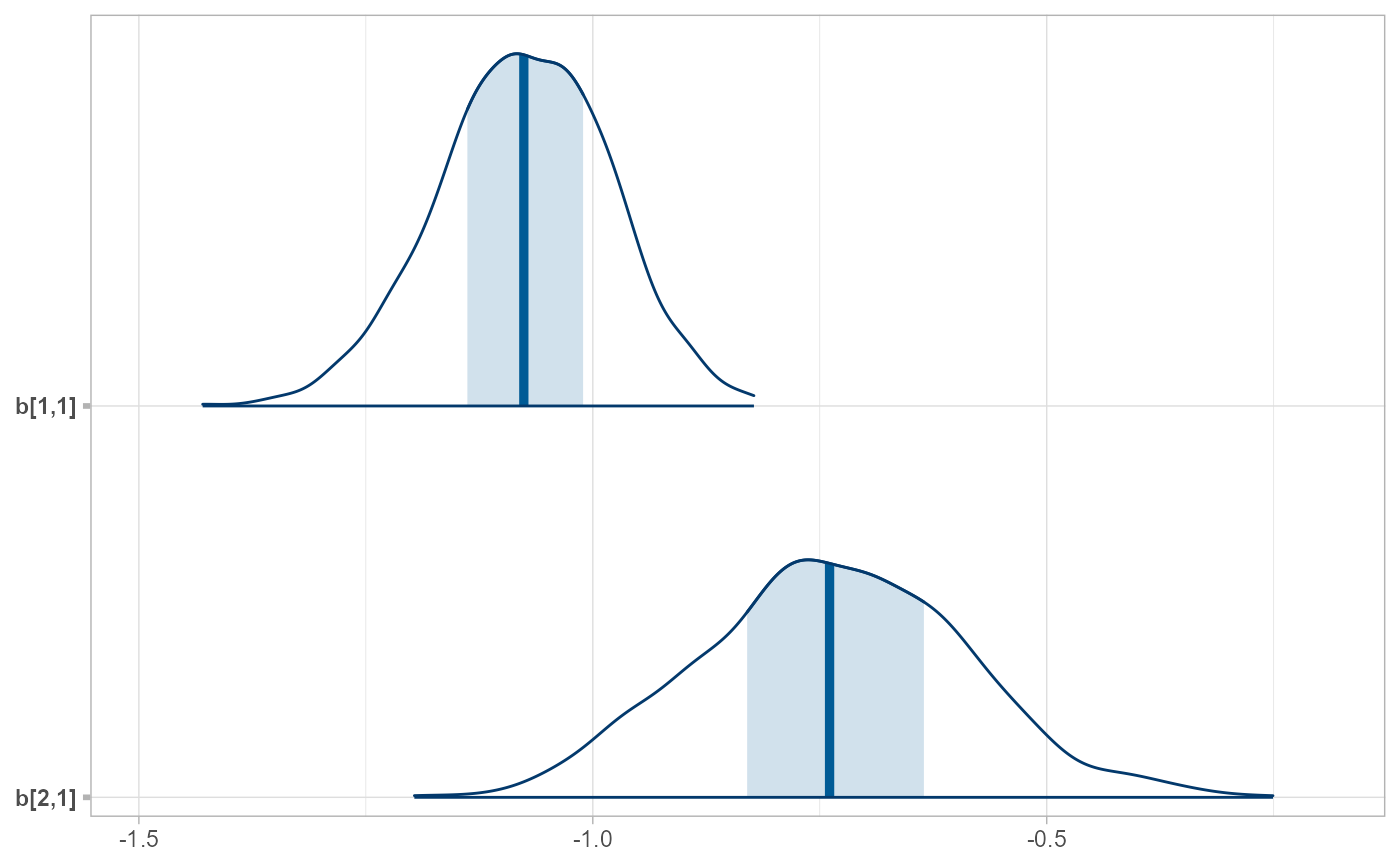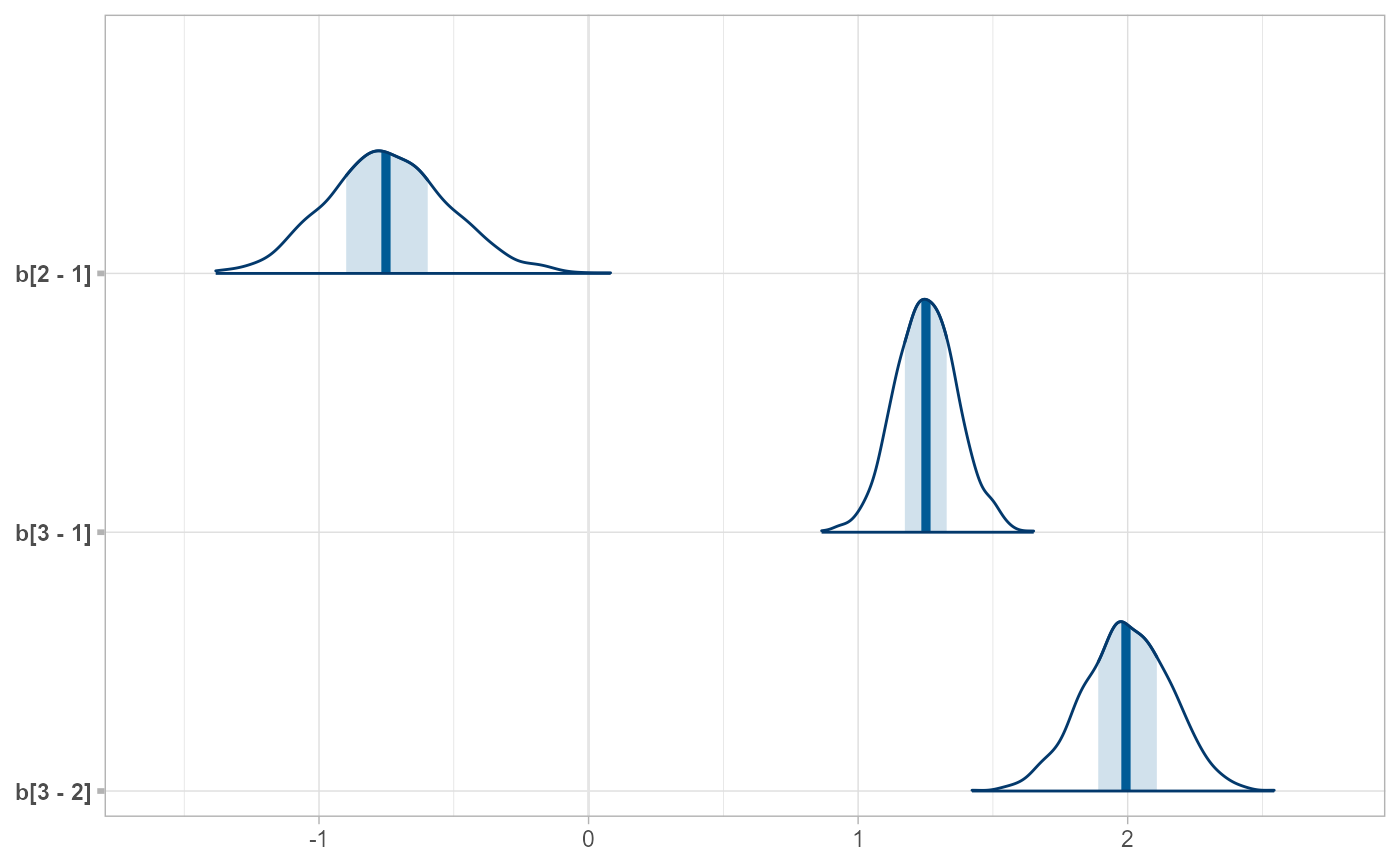Inverse operations of spread_draws() and gather_draws(), giving results that look like tidy_draws().

ungather_draws(
data,
...,
variable = ".variable",
value = ".value",
draw_indices = c(".chain", ".iteration", ".draw"),
drop_indices = FALSE
)

data,
...,
draw_indices = c(".chain", ".iteration", ".draw"),
drop_indices = FALSE
)

## Arguments

data

A tidy data frame of draws, such as one output by spread_draws or gather_draws.

...

Expressions in the form of variable_name[dimension_1, dimension_2, ...]. See spread_draws().

variable

The name of the column in data that contains the names of variables from the model.

value

The name of the column in data that contains draws from the variables.

draw_indices

Character vector of column names in data that should be treated as indices of draws. The default is c(".chain",".iteration",".draw"), which are the same names used for chain, iteration, and draw indices returned by spread_draws() or gather_draws().

drop_indices

Drop the columns specified by draw_indices from the resulting data frame. Default FALSE.

A data frame.

## Details

These functions take symbolic specifications of variable names and dimensions in the same format as spread_draws() and gather_draws() and invert the tidy data frame back into a data frame whose column names are variables with dimensions in them.

spread_draws(), gather_draws(), tidy_draws().

Matthew Kay

## Examples


library(dplyr)

data(RankCorr, package = "ggdist")

# We can use unspread_draws to allow us to manipulate draws with tidybayes
# and then transform the draws into a form we can use with packages like bayesplot.
# Here we subset b[i,j] to just values of i in 1:2 and j == 1, then plot with bayesplot
RankCorr %>%
filter(i %in% 1:2, j == 1) %>%
bayesplot::mcmc_areas()# As another example, we could use compare_levels to plot all pairwise comparisons
# of b[1,j] for j in 1:3
RankCorr %>%